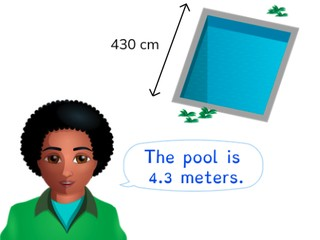Converting from cm and m with decimal numbers

# Converting from cm and m with decimal numbers

Students learn to convert cm and m with decimal numbers.

No account needed.8,000 schools use Gynzy92,000 teachers use Gynzy1,600,000 students use Gynzy

## General

The students learn to convert centimeters to meters and vice versa, for with the number is a decimal number or becomes a decimal number.

## Standards

CCSS.Math.Content.5.MD.A.1

## Learning objective

Students will be able to convert from centimeters (cm) to meters (m) and vice versa with decimal numbers.

## Introduction

On the interactive whiteboard you show a starting number. Then it is shown above whether you must multiply or divide this number by 10, 100, or 1,000. Ask the students what the answer is and then erase the box to show the correct answer. They must then multiply or divide this number by the number given. After the students have given an answer, you can erase the cover again. Discuss all of the problems in this way.

## Instruction

You have two students come to the front of the classroom. Explain that you can measure height and give that in different ways. First one student is measured and their height is given in meters. You then measure the other student, and give their height in centimeters. Next you show an image on the whiteboard of a ruler with a number of bars underneath. The length is given in meters. You can convert this to centimeters. One meter is the same as one hundred centimeters, so seven meters is the same as seven hundred centimeters. After this you add on the extra centimeters. In this way you can also convert from centimeters to meters. Have the students practice converting with help from the images on the interactive whiteboard.
Next you explain, using the diagram of the metric system, how you convert meters to centimeters. For this you take two jumps to the right. You multiply the meters by one hundred. The decimal point moves two places to the right. Discuss this together using a number of other examples. Then you show a number that must be converted to centimeters. There are four possible answers given. Ask the class which answer is correct. Next the students must convert two different numbers without possible answers given. You can also convert centimeters to meters. To do this you take two jumps to the left. So you divide the centimeters by one hundred. The decimal point moves two places to the left. If there is no decimal point in the number, you put one after the last digit and from there you can move it two places to the left. Discuss this together using several examples. After this you show a number that must be converted to meters for which possible answers are given. Ask the class which answer is correct, and then have them convert the other two numbers given. Next you discuss converting in the form of a story problem. If you must convert meters to centimeters, it is a multiplication problem. If you are converting from centimeters to meters, then it is a division problem. Have the students solve a story problem on their own.

To check whether students can convert centimeters to meters and vice versa with decimal numbers, you can ask the following questions:
- How many centimeters is one meter?
- What do you do when you convert centimeters to meters?
- What do you do when you convert meters to centimeters?

## Quiz

The students test their understanding of converting from centimeters and meters with decimal numbers through ten exercises. Some of these exercises have a visual aid, others are abstract problems and others are story problems.

## Closing

You discuss once again with the students that it is useful to be able to convert centimeters to meters and vice versa, because then you can convert to a more logical unit of measurement. Next you show pairs of students on the interactive whiteboard that are each making a statement. Ask the class which of the kids is correct and have them explain how they know this.

## Teaching tips

When students have difficulty with converting centimeters and meters with decimal numbers, you can give them a diagram of the metric system, on which you only have centimeters and meters with an arrow for ÷100 and an arrow for ×100.

## Instruction materials

You may print out a diagram of the metric system.

### The online teaching platform for interactive whiteboards and displays in schools

• Save time building lessons

• Manage the classroom more efficiently

• Increase student engagement